Case Based MCQ Questions

Computer Science - Class 12
Chapter 3 - Stacks

## Millions of computer science students have taken a  course on algorithms and data structures, typically  the second course after the initial one introducing  programming. One of the basic data structures in  such a course is the stack. The stack has a special  place in the emergence of computing as a science,  as argued by Michael Mahoney, the pioneer of the  history of the theory of computing. The Stack can  be used in many computer applications, few are  given below: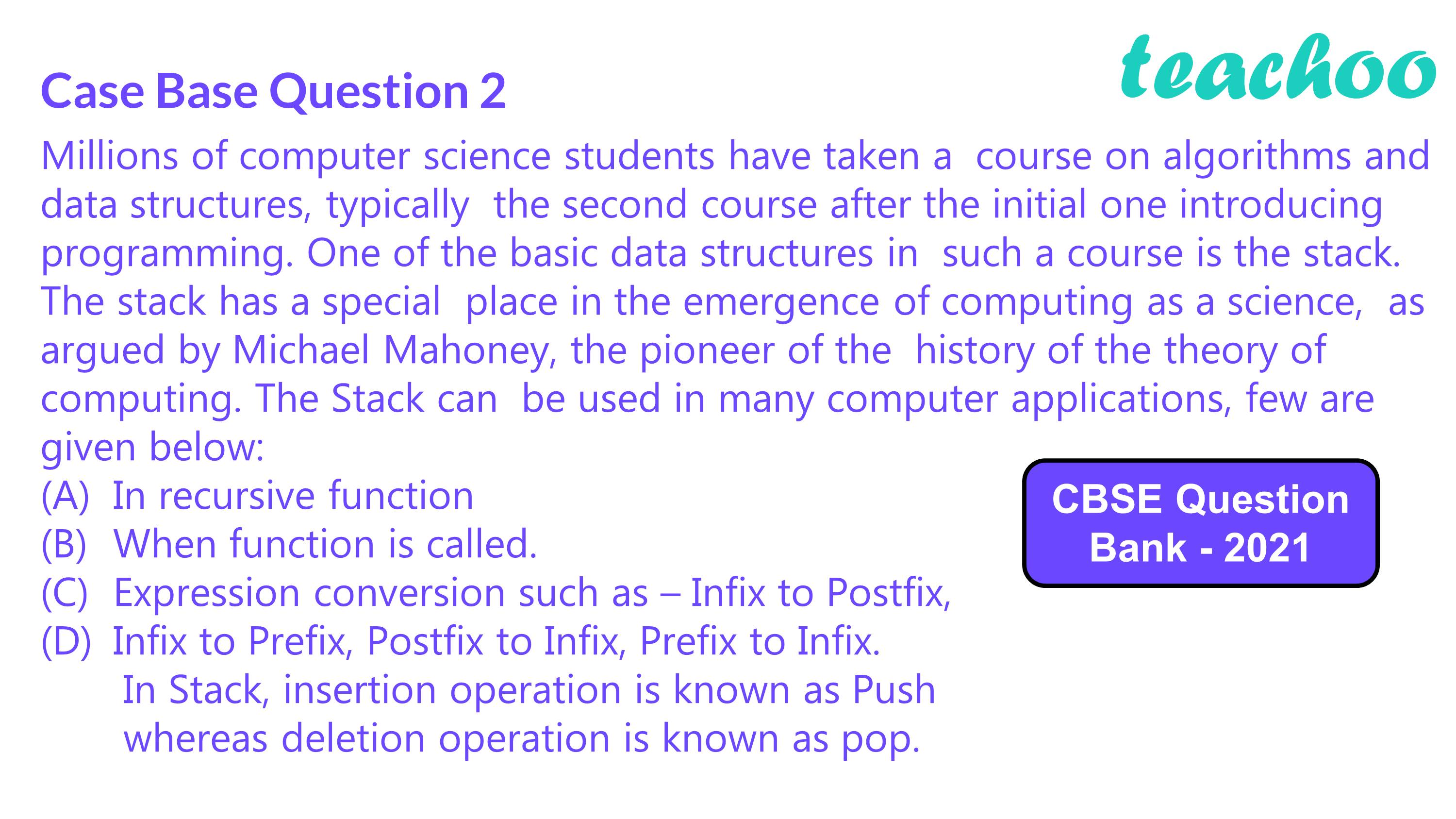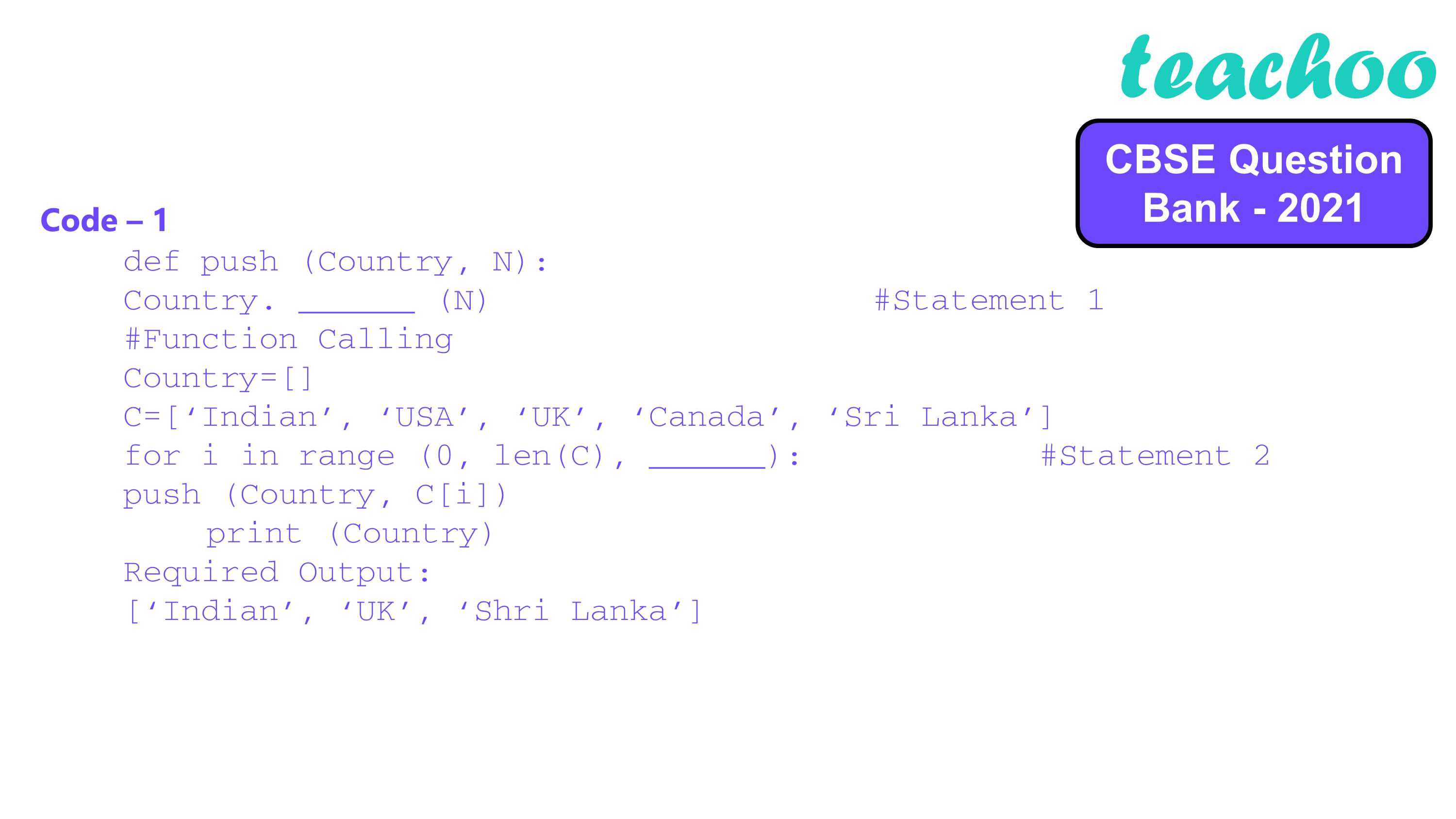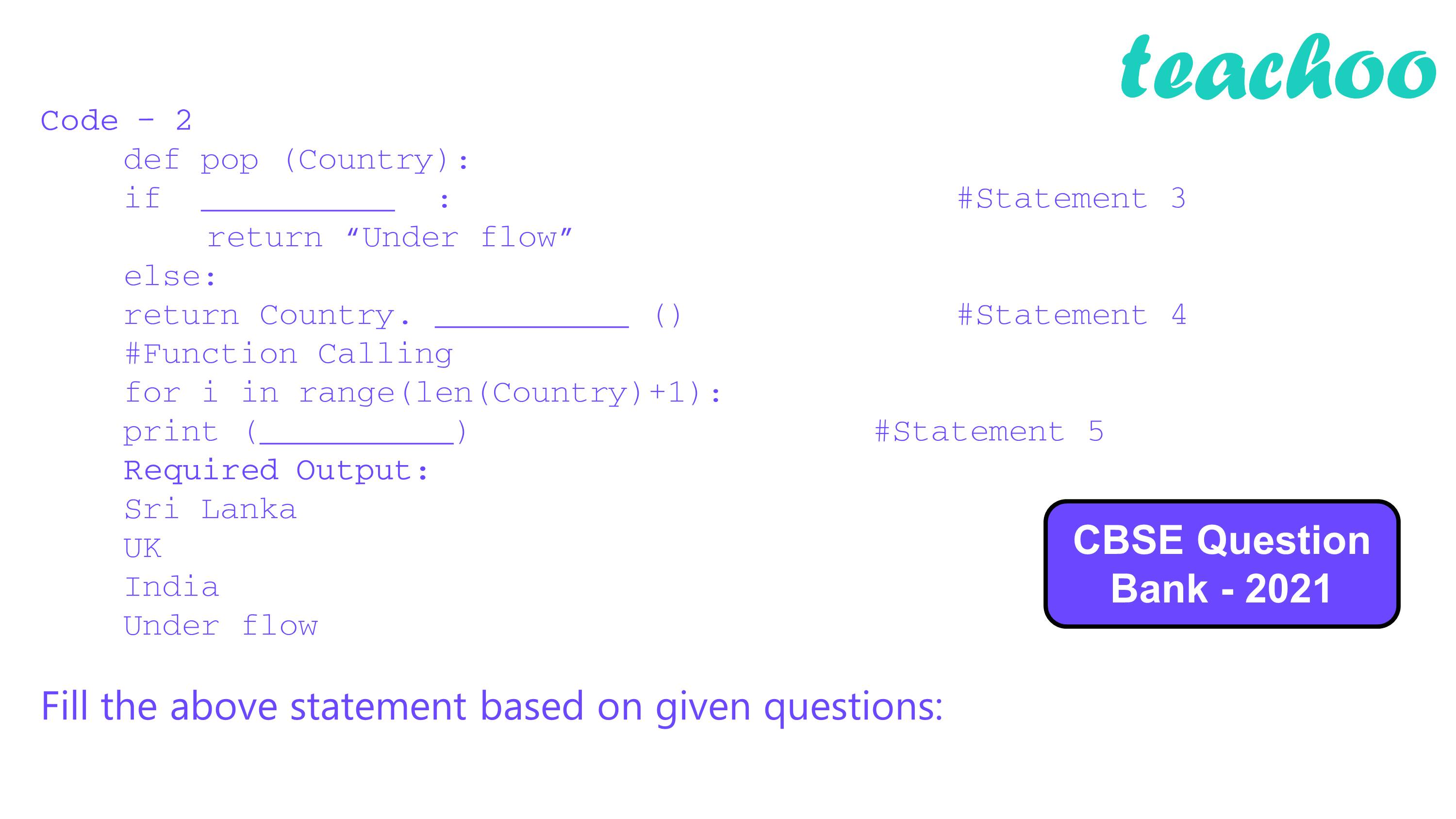##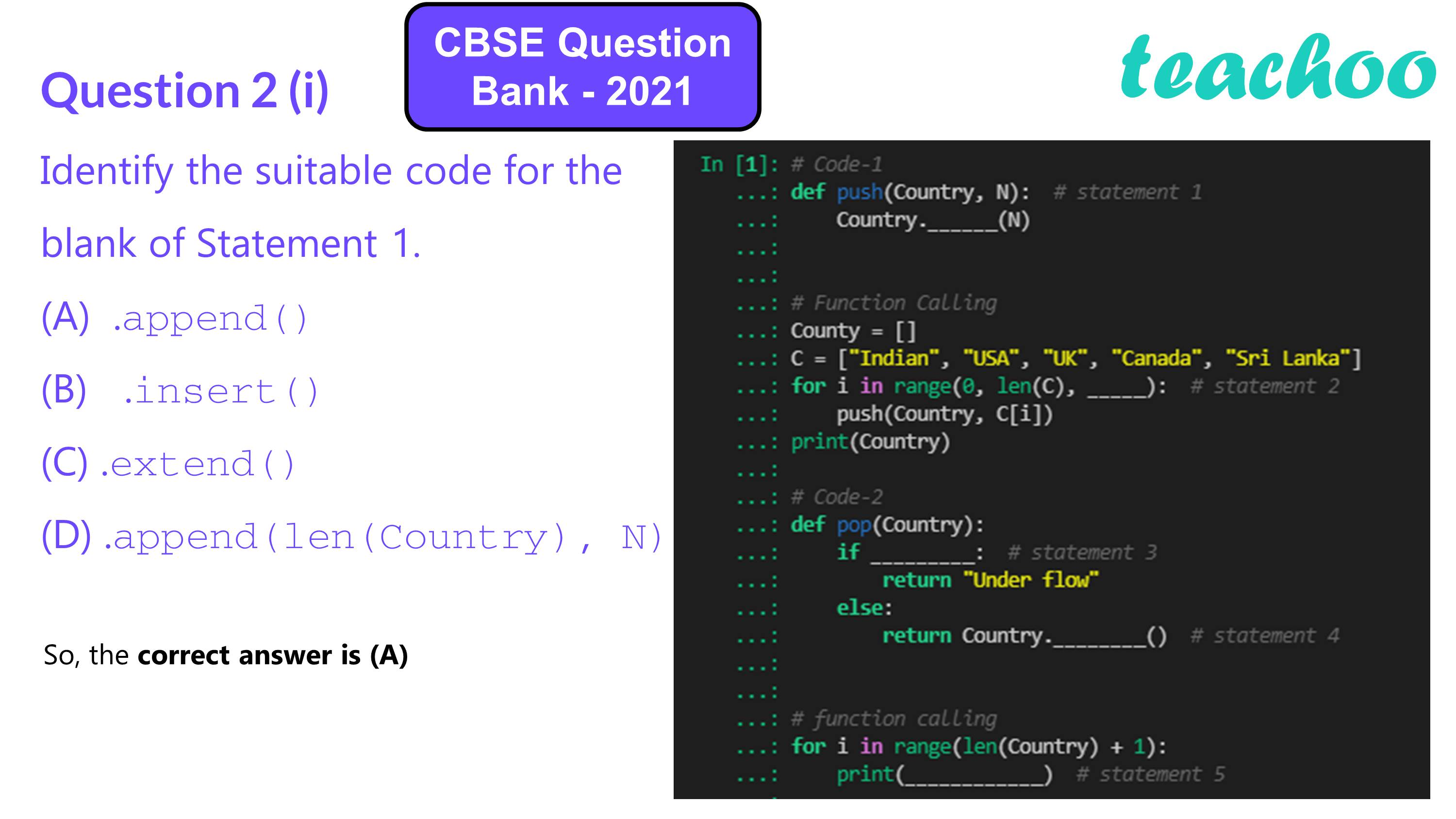(A) .append()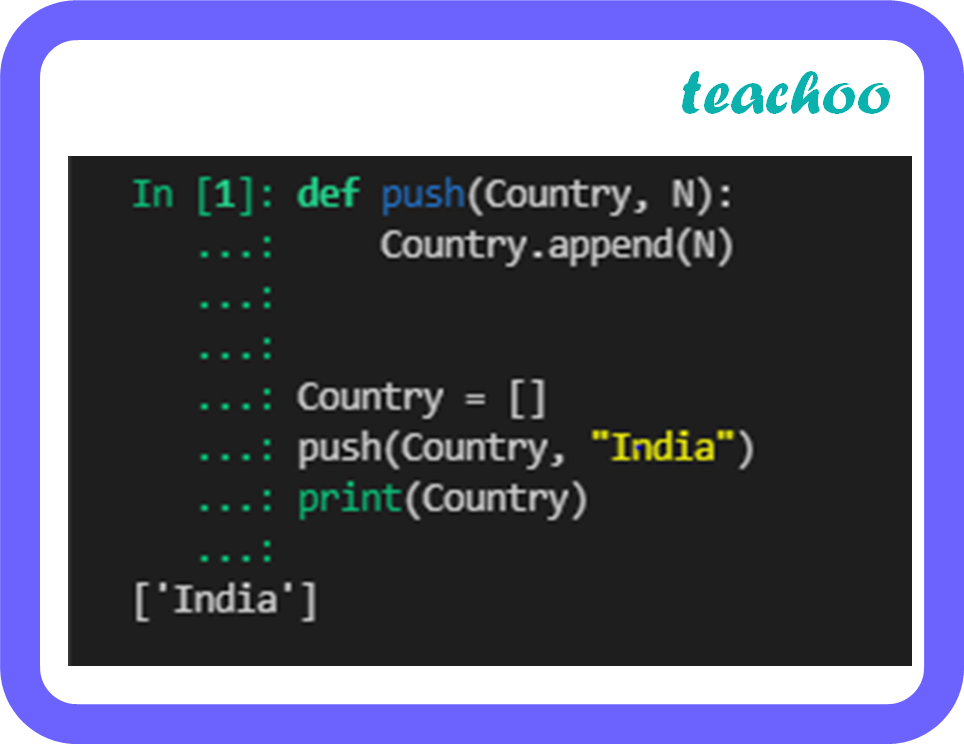(B) .insert()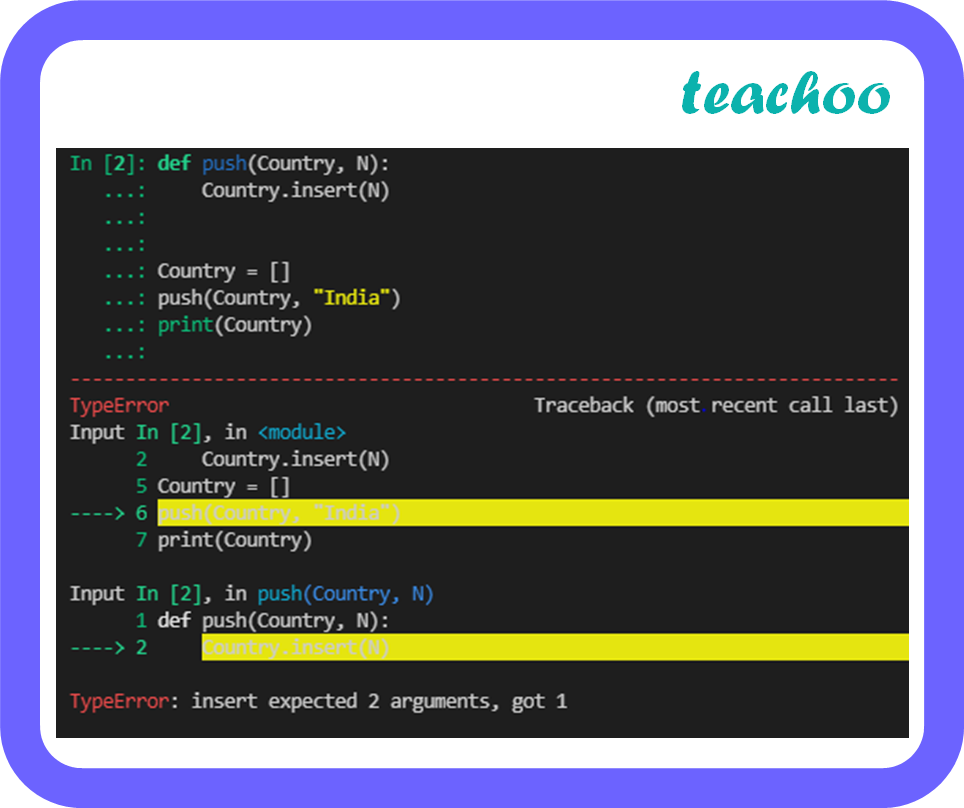(C) .extend()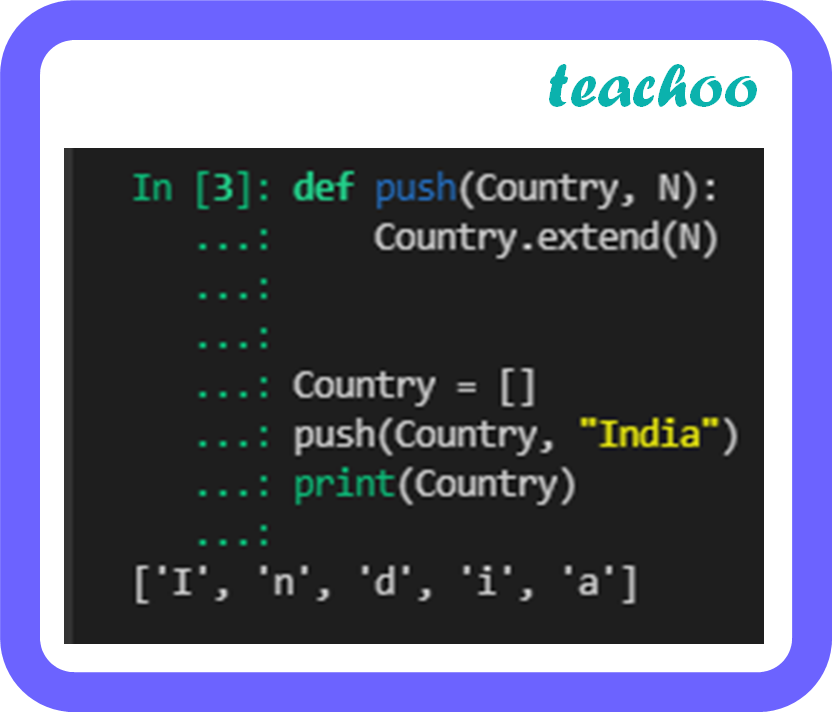(D) .append(Len(Country), N)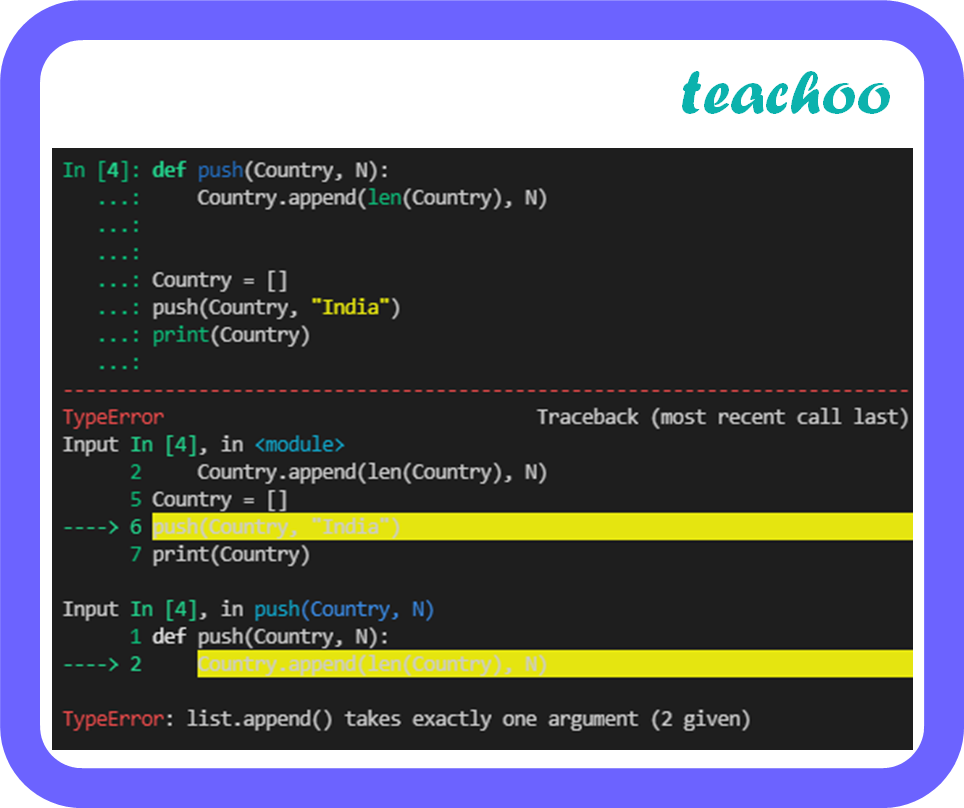## (D) 2

Checking the options

• (A) 3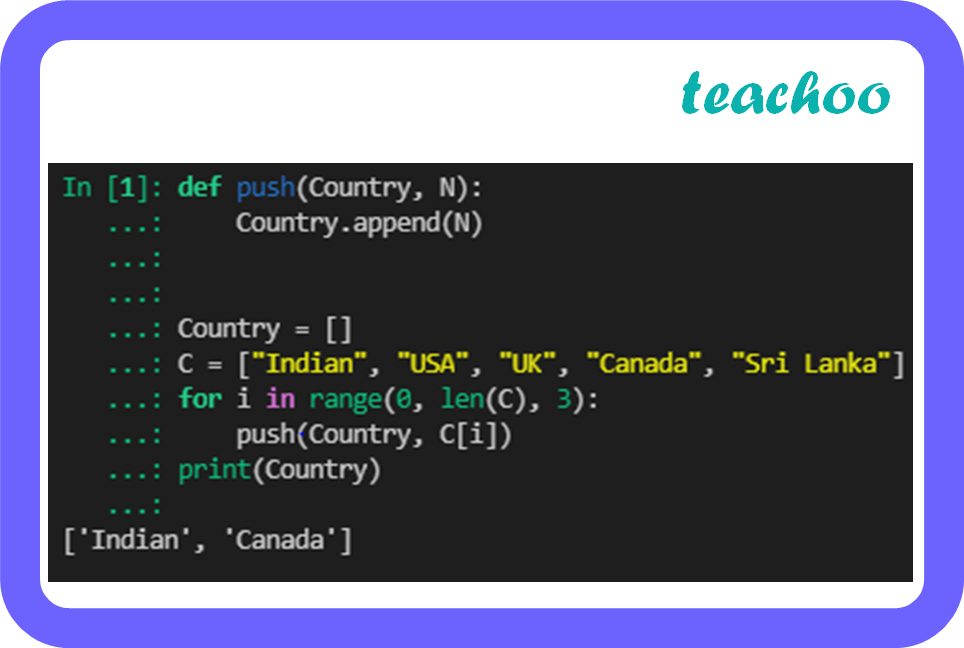(B) 0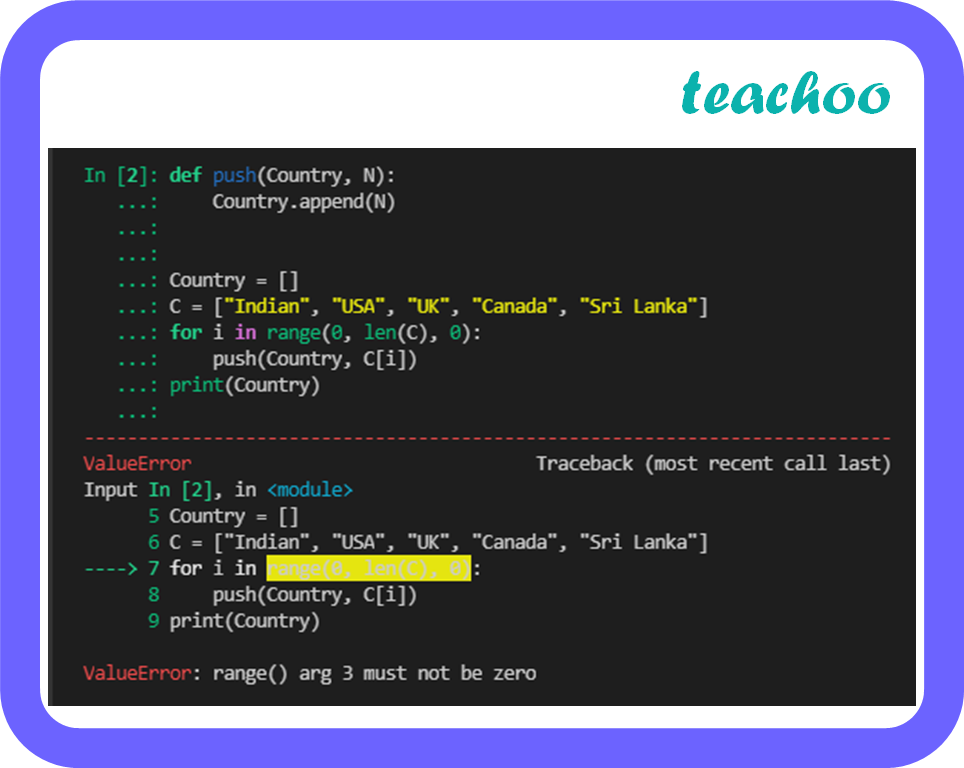(C) –1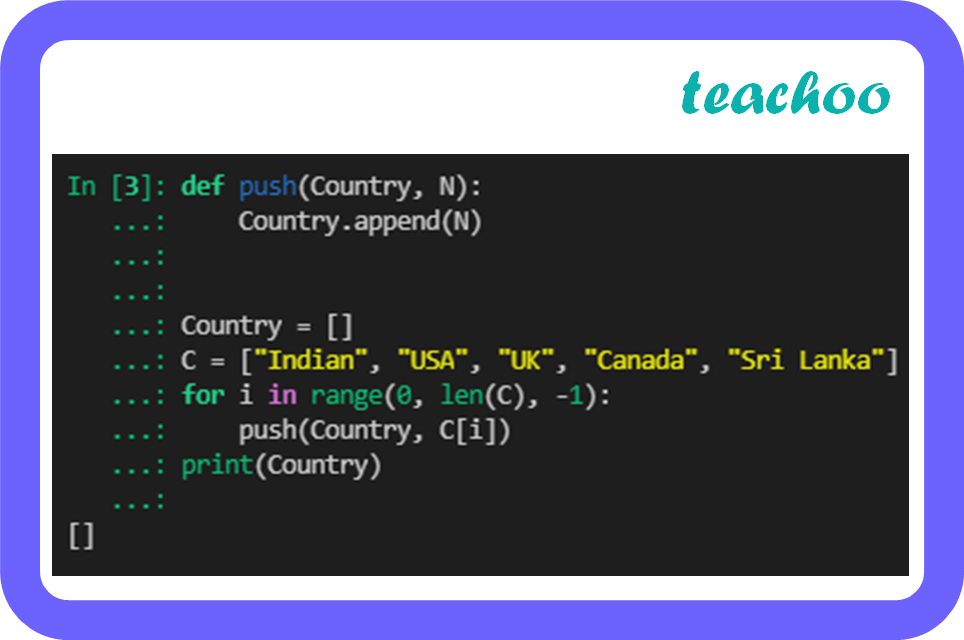(D) 2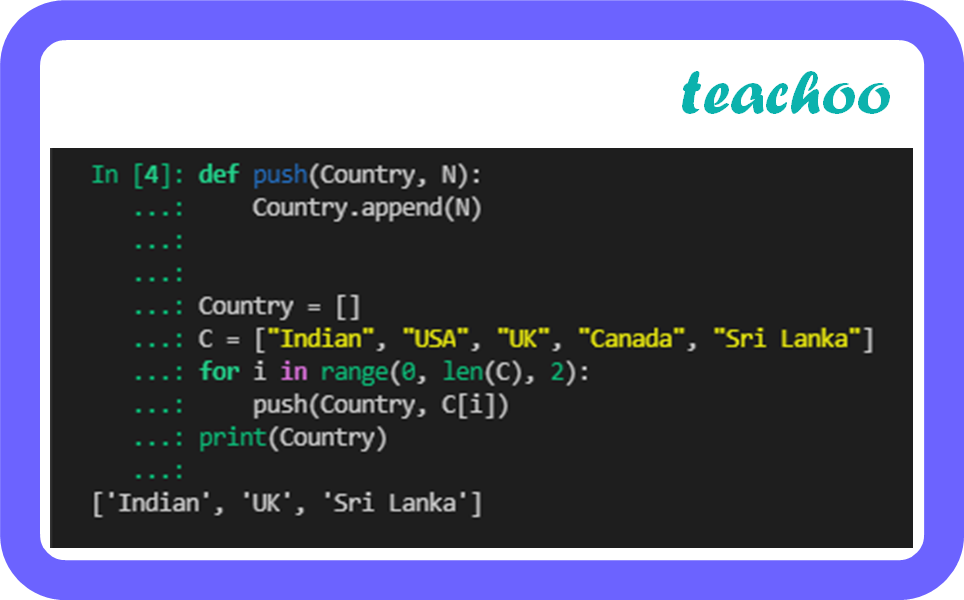So, the correct answer is (D)

## (D) No of the above

Checking the options

• (A) Country=[]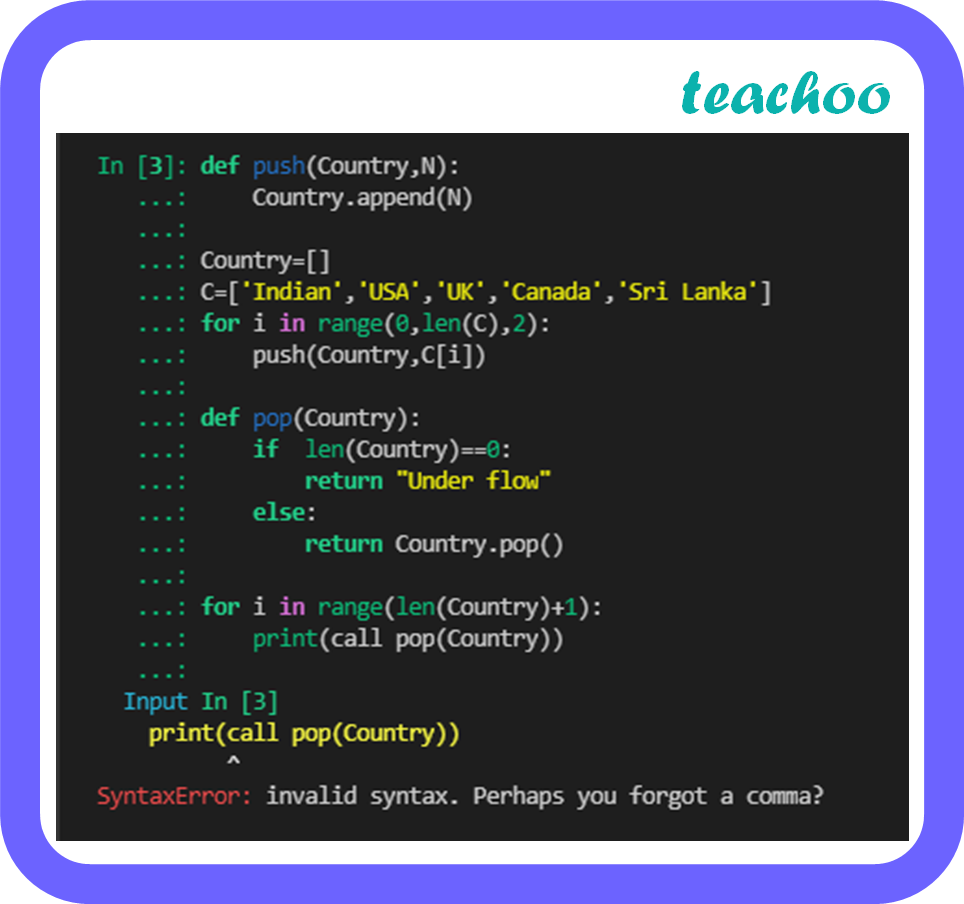(B) Country. is Empty()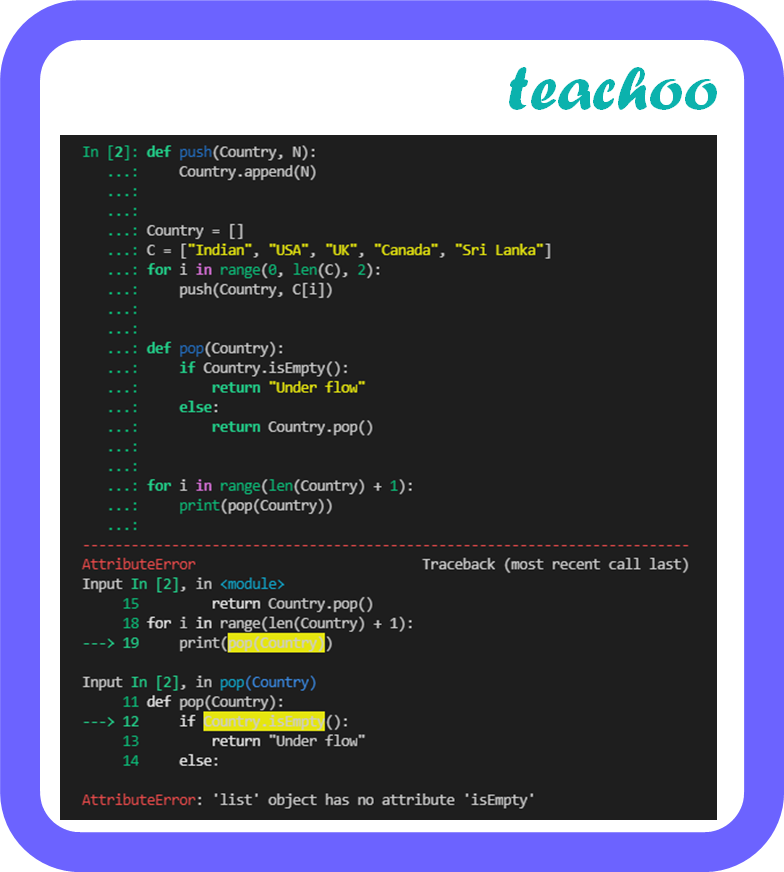(C) len(Country)==0

##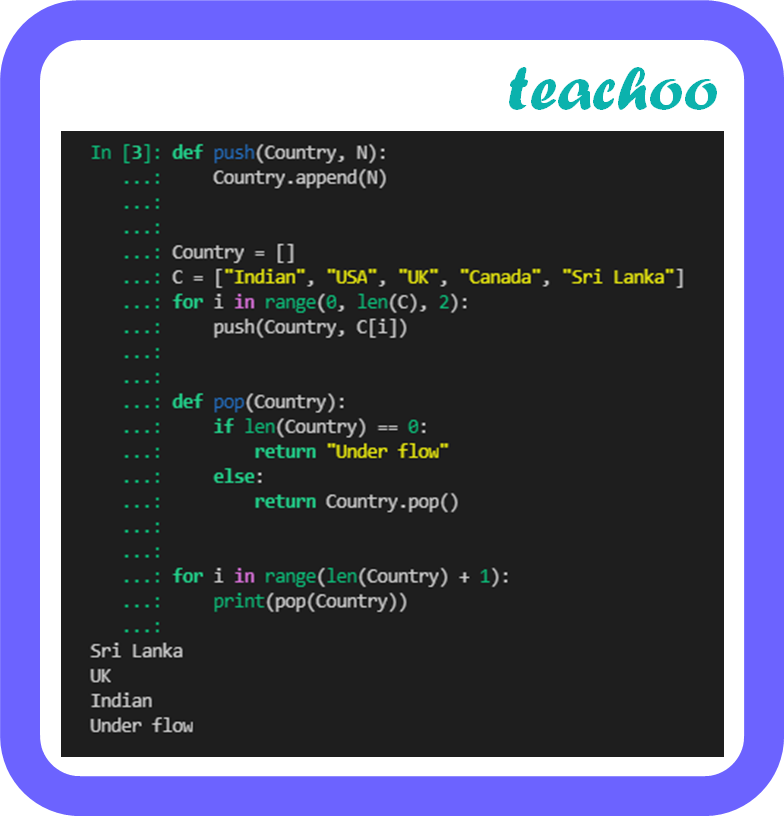(D) No of the above

So, the correct answer is (C)

## (D) Country. delete(1)

Checking the options

• (A) pop(1)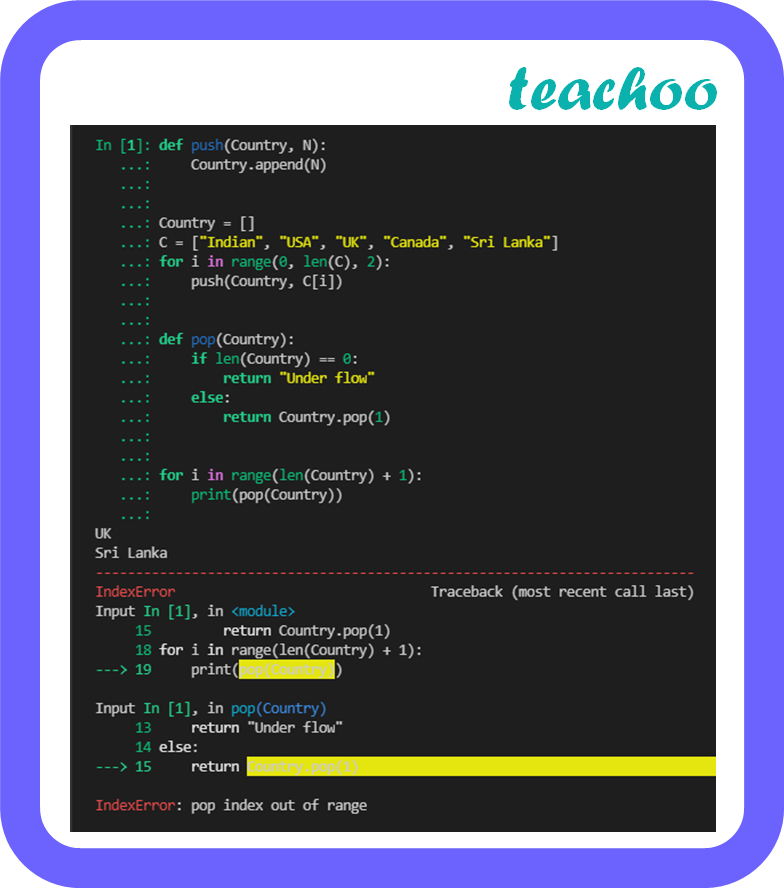(B) pop()

##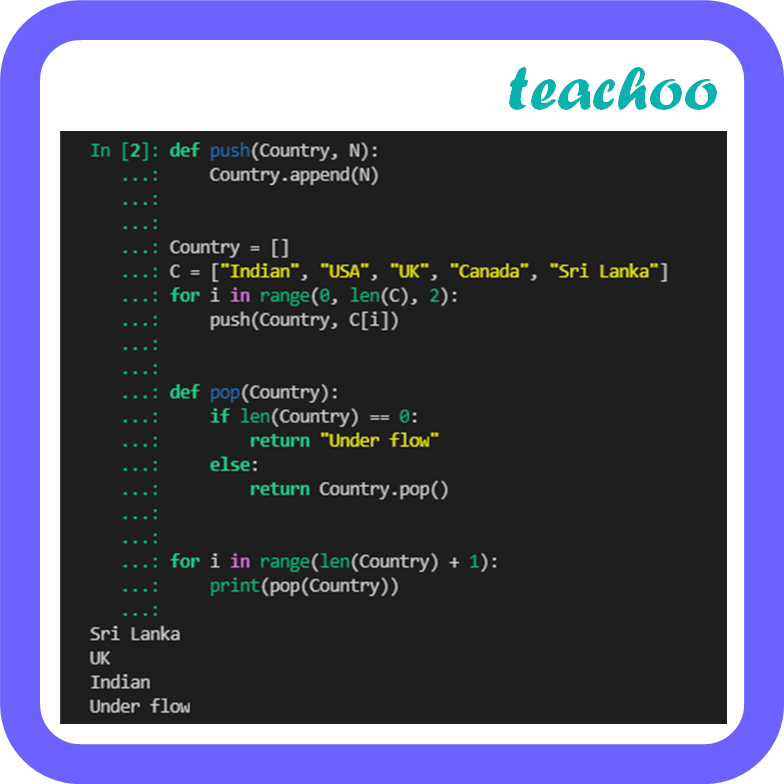(C) del country

##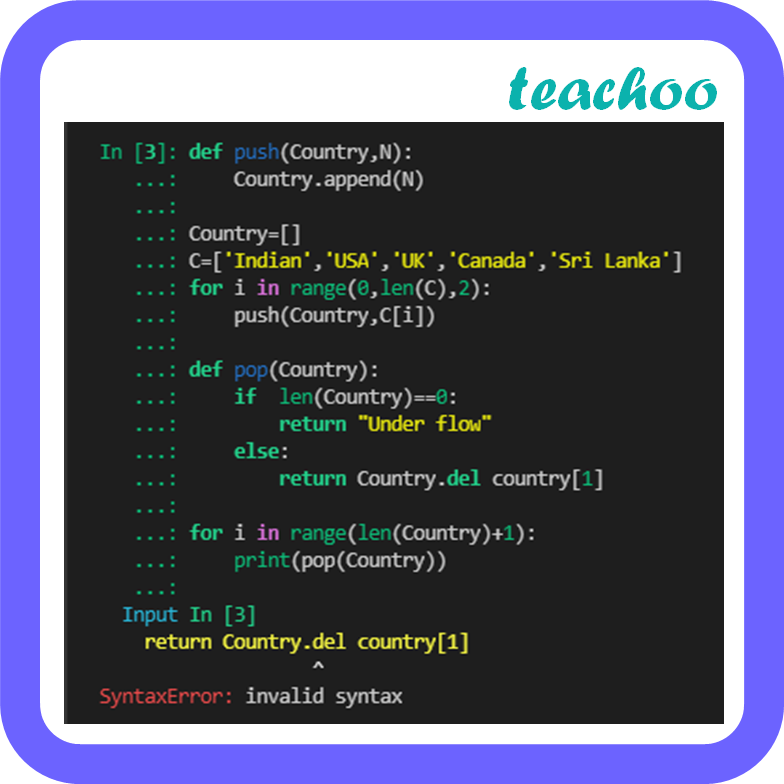(D) Country. delete(1)

##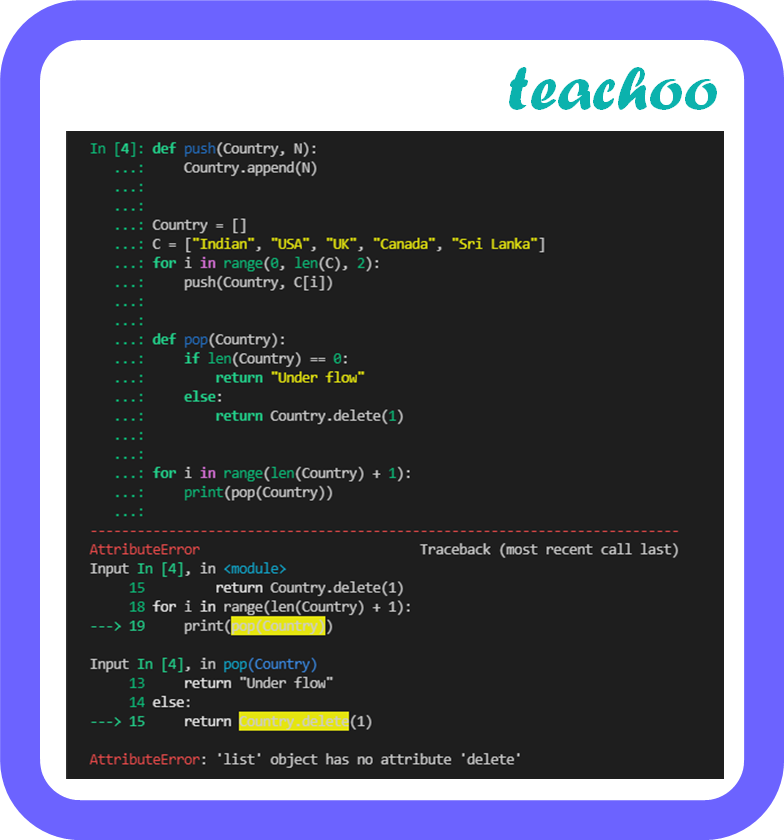So, the correct answer is (B)

## (D) def pop(Country)

Checking the options

• (A) pop(C)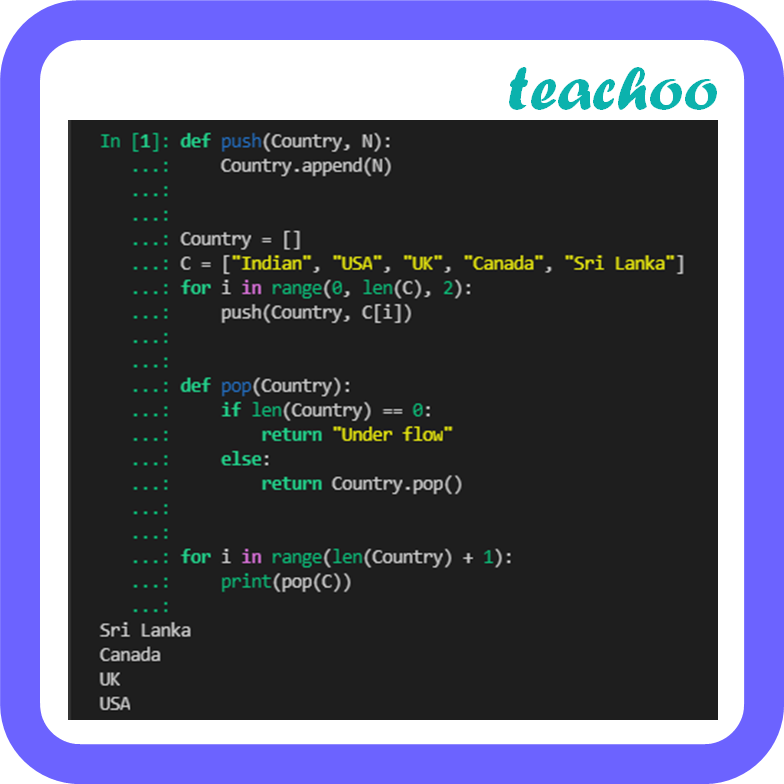(B) pop(Country)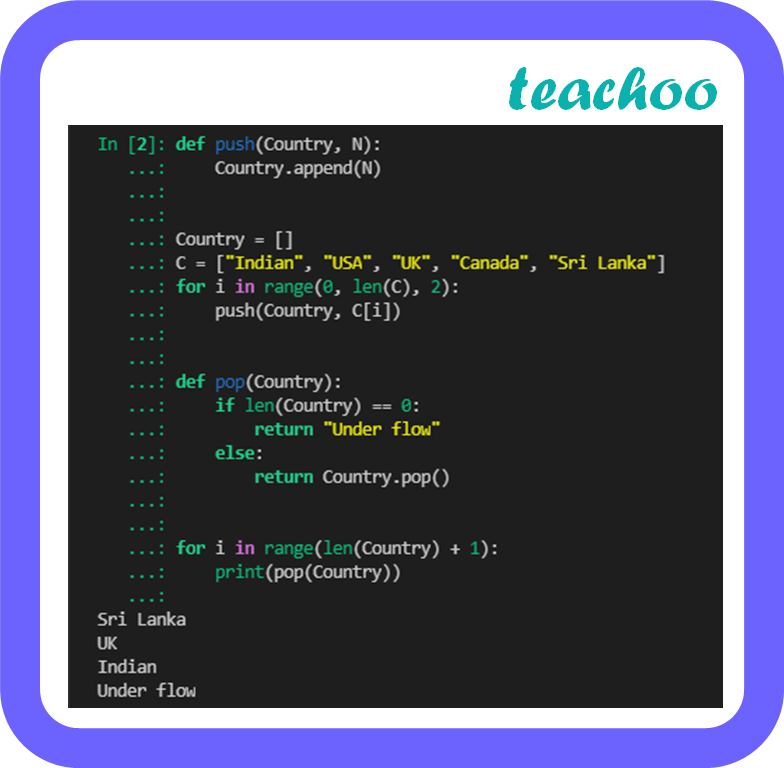(C) call pop(Country)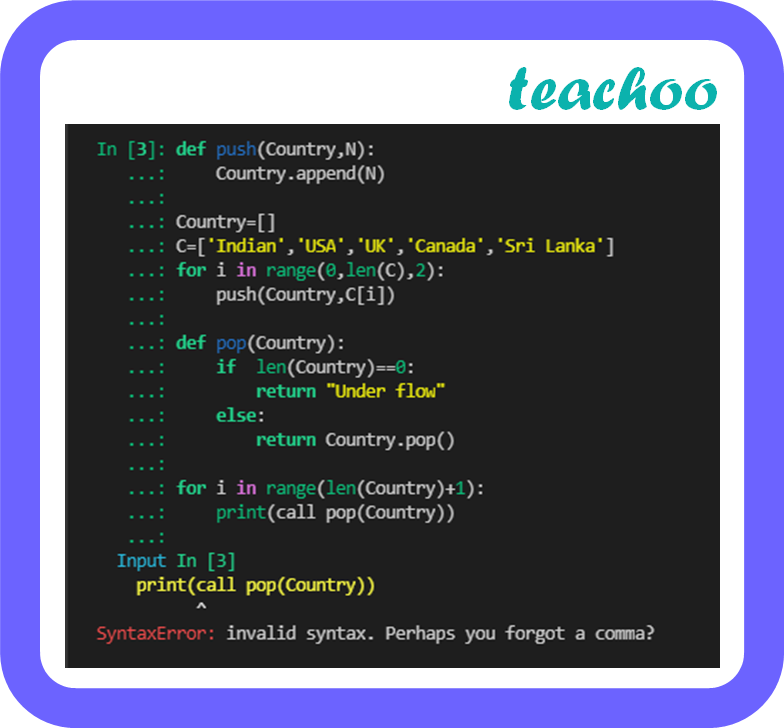(D) def pop(Country)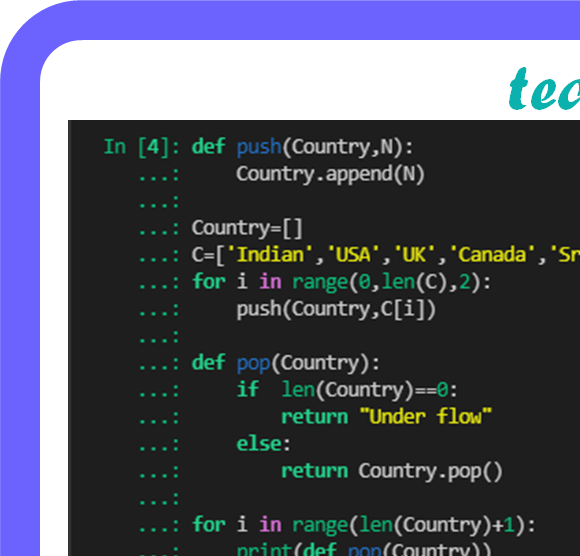So, the correct answer is (B)

Learn in your speed, with individual attention - Teachoo Maths 1-on-1 Class

### Transcript

Case Base Question 2 Millions of computer science students have taken a course on algorithms and data structures, typically the second course after the initial one introducing programming. One of the basic data structures in such a course is the stack. The stack has a special place in the emergence of computing as a science, as argued by Michael Mahoney, the pioneer of the history of the theory of computing. The Stack can be used in many computer applications, few are given below: In recursive function When function is called. Expression conversion such as – Infix to Postfix, Infix to Prefix, Postfix to Infix, Prefix to Infix. In Stack, insertion operation is known as Push whereas deletion operation is known as pop. Code – 1 def push (Country, N): Country. ______ (N) #Statement 1 #Function Calling Country=[] C=[‘Indian’, ‘USA’, ‘UK’, ‘Canada’, ‘Sri Lanka’] for i in range (0, len(C), ______): #Statement 2 push (Country, C[i]) print (Country) Required Output: [‘Indian’, ‘UK’, ‘Shri Lanka’] Code – 2 def pop (Country): if __________ : #Statement 3 return “Under flow” else: return Country. __________ () #Statement 4 #Function Calling for i in range(len(Country)+1): print (__________) #Statement 5 Required Output: Sri Lanka UK India Under flow Fill the above statement based on given questions: (A) .append() (B) .insert() (C) .extend() (D) .append(len(Country), N)